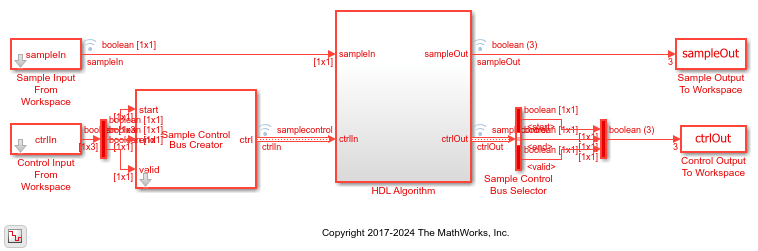# Turbo Encode Streaming Samples

This example shows how to use the LTE Turbo Encoder block to encode data, and how to compare the hardware-friendly design with the results from LTE Toolbox™. The workflow follows these steps:

1. Generate frames of random input samples in MATLAB®.

2. Encode the data using the LTE Toolbox function `lteTurboEncode`.

3. Convert framed input data to a stream of samples and import the stream into Simulink®.

4. To encode the samples using a hardware-friendly architecture, run the Simulink model, which contains the Wireless HDL Toolbox™ block LTE Turbo Encoder.

5. Export the stream of encoded samples to the MATLAB workspace.

6. Convert the sample stream back to framed data, and compare the frames with the reference data.

Generate input data frames. Generate reference encoded data using `lteTurboEncode`.

```rng(0); turboframesize = 40; numframes = 2; txBits = cell(1,numframes); codedData = cell(1,numframes); for ii = 1:numframes txBits{ii} = logical(randi([0 1],turboframesize,1)); codedData{ii} = lteTurboEncode(txBits{ii}); end ```

Serialize input data for the Simulink model. Leave enough time between frames for each frame to be fully encoded before the next one starts. The LTE Turbo Encoder block takes `inframesize` + 16 cycles to complete encoding of a frame.

```inframes = txBits; inframesize = size(inframes{1},1); idlecyclesbetweensamples = 0; idlecyclesbetweenframes = inframesize+16; [sampleIn,ctrlIn] = ... whdlFramesToSamples(inframes, ... idlecyclesbetweensamples, ... idlecyclesbetweenframes); ```

Run the Simulink model. The simulation time equals the number of input samples. Because of the added idle cycles between frames, the streaming input data includes enough cycles for the model to complete encoding of both frames.

```sampletime = 1; samplesizeIn = 1; simTime = size(ctrlIn,1); modelname = 'ltehdlTurboEncoderModel'; open_system(modelname); sim(modelname); ```The Simulink model exports `sampleOut_ts` and `ctrlOut_ts` back to the MATLAB workspace. Deserialize the output samples, and compare the framed data to the reference encoded frames.

The output samples of the LTE Turbo Encoder block are interleaved with the parity bits.

Hardware-friendly output: `S_1 P1_1 P2_1 S2 P1_2 P2_2 ... Sn P1_n P2_n`

LTE Toolbox output: `S_1 S_2 ... S_n P1_1 P1_2 ... P1_n P2_1 P2_2 ... P2_n`

Reorder the samples using the interleave option of the `whdlSamplesToFrames` function. Compare the reordered output frames with the reference encoded frames.

```sampleOut = sampleOut'; interleaveSamples = true; outframes = whdlSamplesToFrames(sampleOut(:),ctrlOut,[],interleaveSamples); fprintf('\nLTE Turbo Encoder\n'); for ii = 1:numframes numBitsDiff = sum(outframes{ii} ~= codedData{ii}); fprintf([' Frame %d: Behavioral and ' ... 'HDL simulation differ by %d bits\n'],ii,numBitsDiff); end ```
```Maximum frame size computed to be 132 samples. LTE Turbo Encoder Frame 1: Behavioral and HDL simulation differ by 0 bits Frame 2: Behavioral and HDL simulation differ by 0 bits ```